# Perpendicular forces acting in x and y directions

• Bolter
Bolter
Homework Statement
Finding additional force f, that balances Fx and Fy
Relevant Equations
Resolving forces
Hi everyone!

So I got a question shown below here and I’m struggling in what the question is trying to say here and what it wants me to give?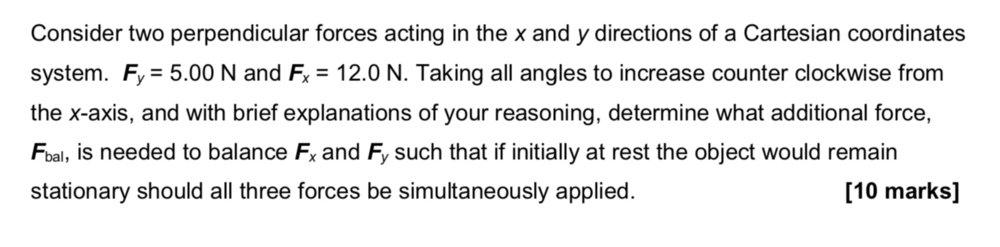Here is what I done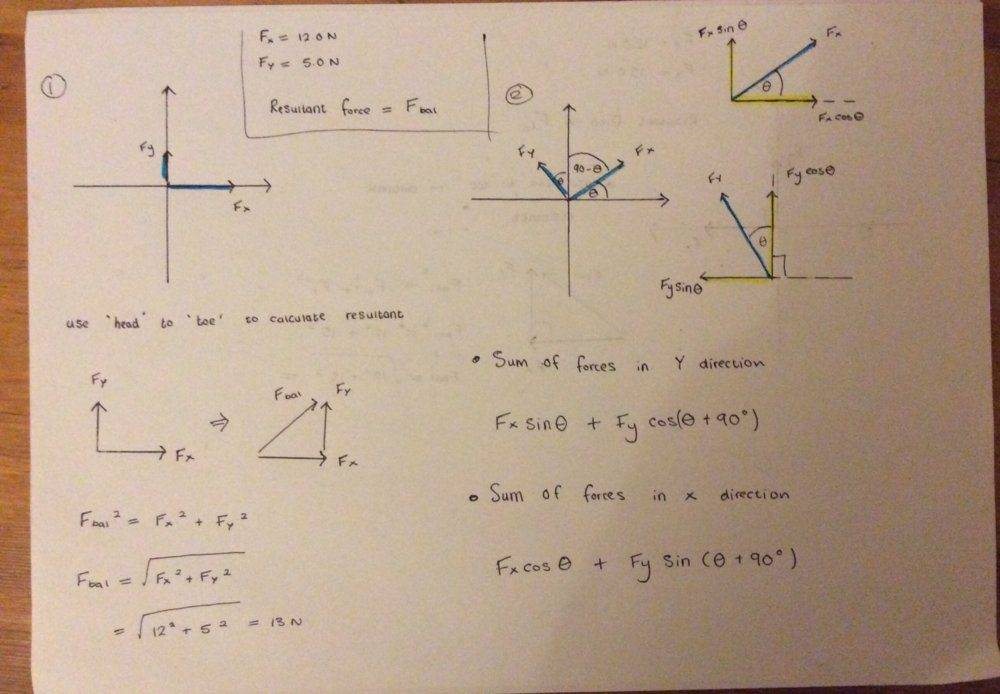For when resolving the individual x and y component of forces, my angle has always been measured counter clockwise from the positive x-axis initially.

Any help would be really great! Thanks

Mentor
Homework Statement:: Finding additional force f, that balances Fx and Fy
Homework Equations:: Resolving forces

So I got a question shown below here and I’m struggling in what the question is trying to say here and what it wants me to give?
You've got two existing forces acting on some object at the origin. Those two forces will sum to some resultant force. (what angle will this resultant force make with the + x-axis?)

Now, what third force can you apply in order that the sum of all forces will be zero? In other words, what new force will you need to apply to balance the resultant above?

Bolter
You've got two existing forces acting on some object at the origin. Those two forces will sum to some resultant force. (what angle will this resultant force make with the + x-axis?)

Now, what third force can you apply in order that the sum of all forces will be zero? In other words, what new force will you need to apply to balance the resultant above?

For the first case, when the 5N force is acting directly along the y-axis and 12N force acting directly along the x axis. Then the angle between the positive x-axis and resultant force is 22.6 degrees from using basic trig.

For the second case, I believe both forces get rotated by some angle theta counter clockwise from the positive x axis. Thus I had tried to resolve both forces into its horizontal and vertical components again leading me to get 4 smaller orthogonal forces. I then grouped the horizontal and vertical components separately. To find this third balancing force, I used Pythagoras. I had left this resultant force in abstract form as the theta angle is unknown here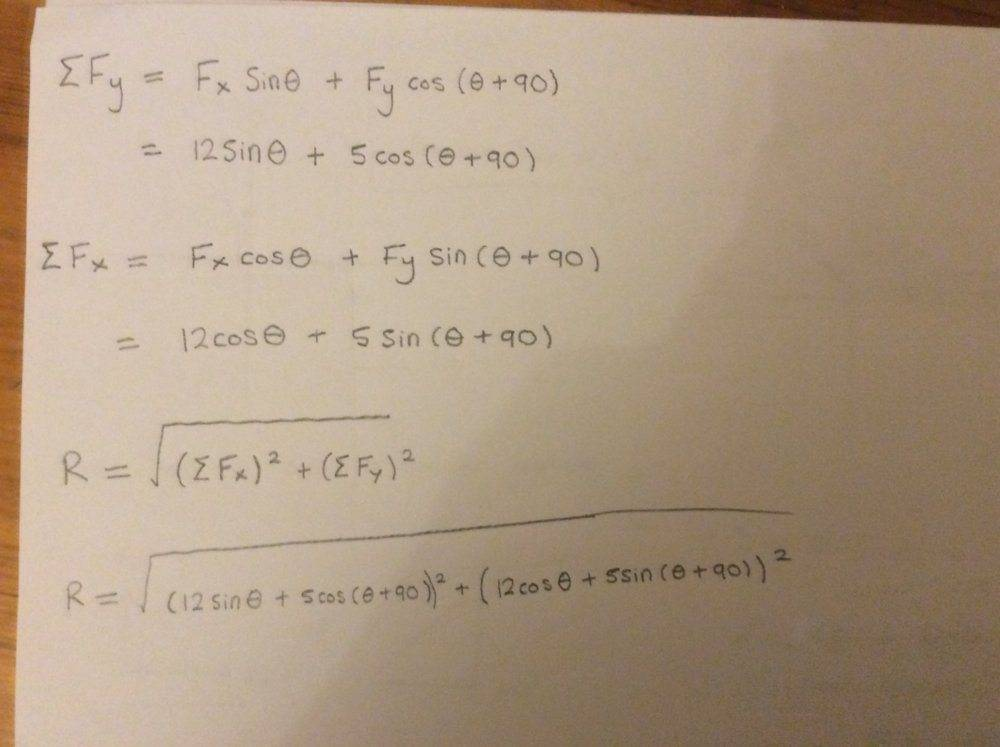Mentor
For the second case, I believe both forces get rotated by some angle theta counter clockwise from the positive x axis.
No, no need to rotate those forces. They retain their original orientation. You simply have to add another force to balance their resultant.

Draw your original forces and form the resultant: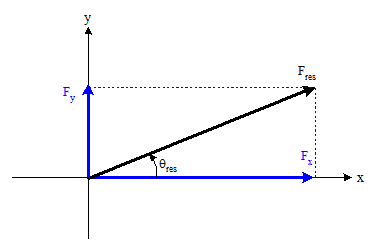Now redraw showing only the resultant (remove the original two forces as the resultant does the same thing as those two original forces). Now, what force would you need to apply to cancel out that resultant?

Bolter
No, no need to rotate those forces. They retain their original orientation. You simply have to add another force to balance their resultant.

Draw your original forces and form the resultant:

View attachment 254842

Now redraw showing only the resultant (remove the original two forces as the resultant does the same thing as those two original forces). Now, what force would you need to apply to cancel out that resultant?

I see now. So to add an additional force that will counteract that resultant would be to apply a force that is of the same magnitude as the resultant but in the opposite direction to the resultant. So the force extra force that should be exerted on the object is a 13N force which is 202.6 degrees from the +ve x axis. So I guess this ensures that when all the forces are applied, including the extra force, new resultant comes out as zero and the object stays stationary.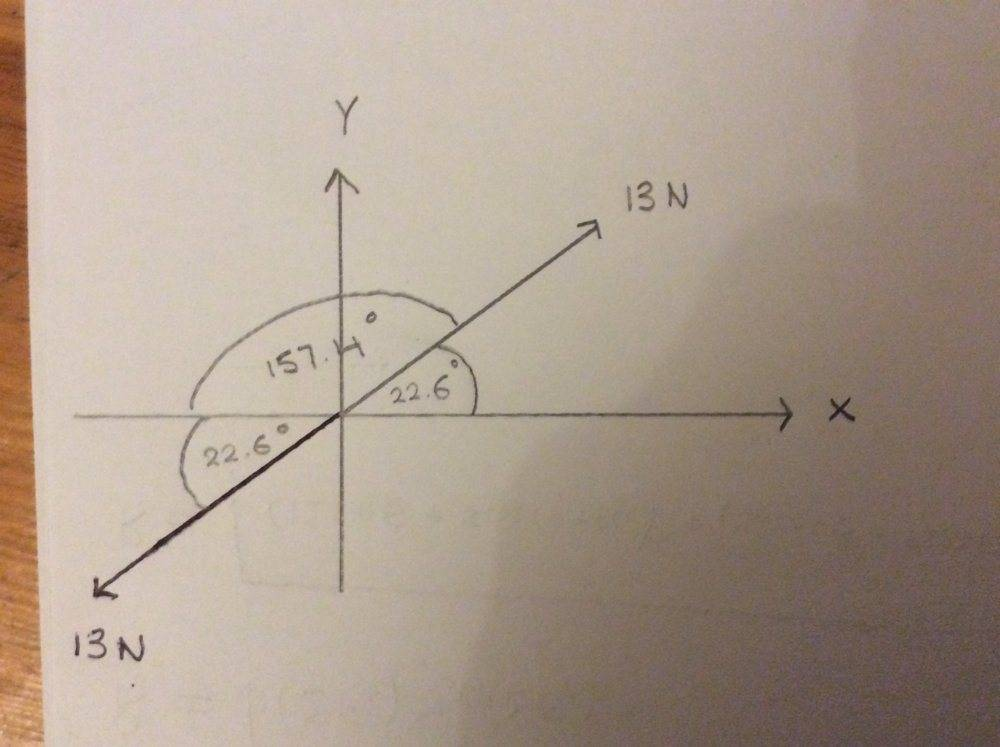Mentor
Right! Although I would likely look at the angles like this: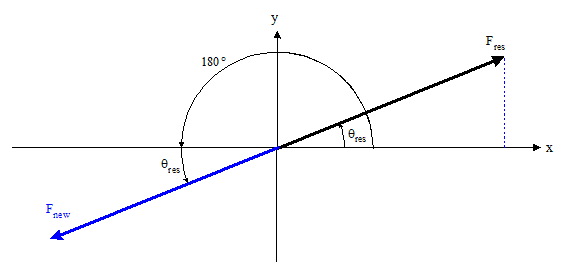Usually we like to see angle values in the range ##-180\deg \le \theta \le 180 \deg##, so you can also specify the angle from the positive x-axis as a negative value going clockwise from the x-axis to the new force in the third quadrant (shown in red below):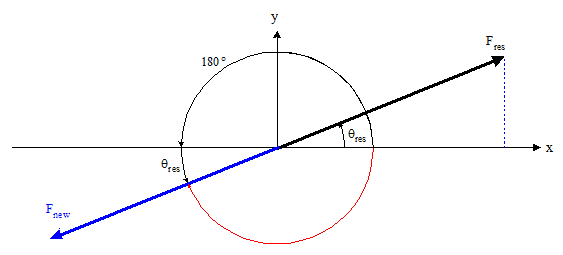•Bolter
Bolter
Ahh so preferably the angle is -180 + 22.6 = -157.4 degrees. Which is noted to be the angle measured from the positive x axis, going in a clockwise direction. Got it•gneill
lightlightsup
Isn't the answer simply: ##\vec{F}_{bal} = -F_x\hat{x} + -F_y\hat{y}##?

Mentor
Isn't the answer simply: ##\vec{F}_{bal} = -F_x\hat{x} + -F_y\hat{y}##?
In this case, yes it is.